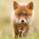1177 görüntülenme
1177
EXPERIMENTAL:
Measures the volatility range of current price range, can help detect squeezes as well explosive moves
```//@version=2
study(title='[RS]Volatility Explosive Measure V0')
f_bullish_sequence()=>
_output = 0
_range = close - open
_doji = _range == 0
_up_bar = _range > 0 or _doji and _range > 0
_counter = barssince(not _up_bar)
if (_up_bar)
for _i = 0 to max(0, _counter - 1)
_output := _output + _range[_i]
_output

f_bearish_sequence()=>
_output = 0
_range = close - open
_doji = _range == 0
_up_bar = _range < 0 or _doji and _range < 0
_counter = barssince(not _up_bar)
if (_up_bar)
for _i = 0 to max(0, _counter - 1)
_output := _output + _range[_i]
_output

bull_seq = f_bullish_sequence()
bear_seq = f_bearish_sequence()

length = input(10)
bull_ma = ema(bull_seq, length)
bear_ma = ema(bear_seq, length)
width = bull_ma - bear_ma

plot(width, color=color(black, 0), style=area)
// plot(bull_seq, color=color(lime, 0), style=columns)
// plot(bear_seq, color=color(red, 0), style=columns)
// plot(bull_ma, color=color(purple, 25), style=area)
// plot(bear_ma, color=color(purple, 25), style=area)
```sk12722
the higher the value the bigger the explosive movement, lower less movement generally peaks would be tops/bottoms but not always, as a top/bottom may not occur always at the end of the explosive move
Cevap Gönder